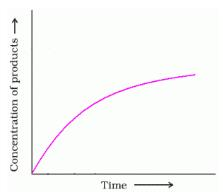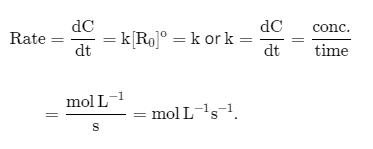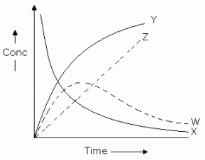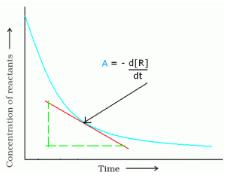Courses

# Test: Rate of Chemical Reaction

## 10 Questions MCQ Test Chemistry for JEE | Test: Rate of Chemical Reaction

Description
This mock test of Test: Rate of Chemical Reaction for JEE helps you for every JEE entrance exam. This contains 10 Multiple Choice Questions for JEE Test: Rate of Chemical Reaction (mcq) to study with solutions a complete question bank. The solved questions answers in this Test: Rate of Chemical Reaction quiz give you a good mix of easy questions and tough questions. JEE students definitely take this Test: Rate of Chemical Reaction exercise for a better result in the exam. You can find other Test: Rate of Chemical Reaction extra questions, long questions & short questions for JEE on EduRev as well by searching above.
QUESTION: 1

### A foreign substance that increase the speed of a chemical reaction is called

Solution:

Catalyst: Substances which alter  the rate of a chemical reaction and themselves remain chemically and quantitatively unchanged  after the reaction are known as catalysts and the phenomenon is known as catalysis.

QUESTION: 2

### . What will be the value of instantaneous rate of reaction from the graph?Solution:

If you make a graph of concentration of reactant vs time, the instantaneous reaction rate at a given time is the slope of the tangent line at that point in time. It is also the value of the rate law at a specific concentration (dA/dt is rate = k [A]).

QUESTION: 3

### The rate of a chemical reaction doubles for every 10°C rise of temperature. If the temperature is raised by 50°C, the rate of the reaction increases by about  [AIEEE 2011]

Solution:

For 2times=10°c.
for 4times=20°c.
for 6times=30°c.
for 8times=40°c.
and for 10times=50°c.

For every 10°C rise of temperature, the rate is doubled. Thus, the temperature coefficient of the reaction = 2

When temperature is increased by 50°, rate becomes =2(50/10) = 25 times = 32 times

QUESTION: 4

Chemical substances speeding up rate of chemical reaction is called as

Solution:

A catalyst is a substance that can be added to a reaction to increase the reaction rate without getting consumed in the process. Catalysts typically speed up a reaction by reducing the activation energy or changing the reaction mechanism. Enzymes are proteins that act as catalysts in biochemical reactions.

QUESTION: 5

Chemical substances speeding up the rate of chemical reaction is called as

Solution:

A catalyst is a substance that speeds up the rate of the reaction without being consumed by the reaction itself. When a catalyst is added, the activation energy is lowered because the catalyst provides a new reaction pathway with lower activation energy.

QUESTION: 6

The rate constant of  zero-order reactions has the unit

Solution:QUESTION: 7

For the reaction A + B → C+ D . The variation of the concentration of the products is given by curveSolution:

For the reaction, A+B⟶C+D, the  variation of the concentration of the products is given by the curve Y.
Initially, the product concentration is 0, then it gradually rises and reaches a maximum value. After that it remains constant.

This behavior is represented by the curve Y.

QUESTION: 8

The term  -dx/dt  in the rate expression refers to the:

Solution:

The correct answer is option C
The termin the rate expression refers to the instantaneous rate of the reaction.
It is the ratio of the decrease in the concentration of a reaction to the time taken for this decrease.

QUESTION: 9

Instantaneous reaction expresses

Solution:

The correct answer is Option A.

The instantaneous rate is the rate of a reaction at any particular point in time, a period of time that is so short that the concentrations of reactants and products change by a negligible amount.

QUESTION: 10

Identify A in the given diagram.Solution:

The correct answer is Option D
The instantaneous rate is the rate of a reaction at any particular point in time, a period of time that is so short that the concentrations of reactants and products change by a negligible amount. The initial rate is the instantaneous rate of reaction as it starts (as the product just begins to form).